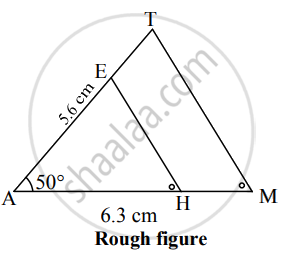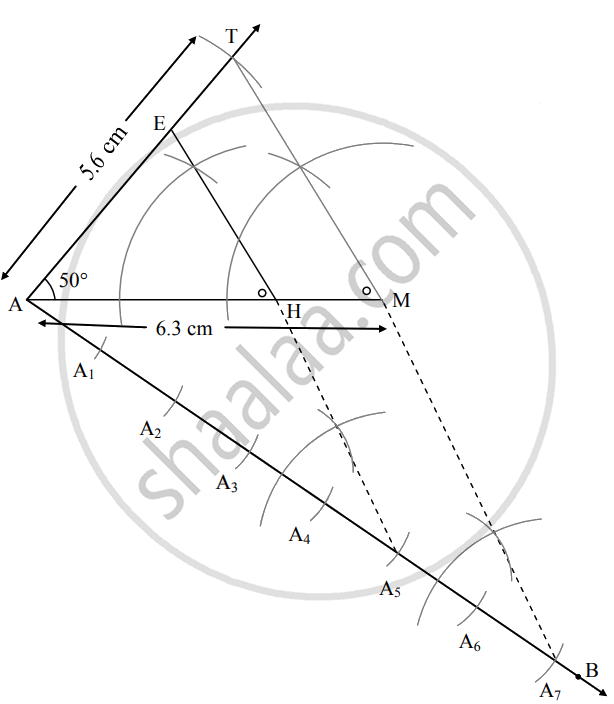# ∆Amt ~ ∆Ahe. in ∆Amt, Am = 6.3 Cm, ∠Tam = 50°, at = 5.6 Cm. a M a H = 7 5 . Construct ∆Ahe. - Geometry

Sum

∆AMT ~ ∆AHE. In ∆AMT, AM = 6.3 cm, ∠TAM = 50°, AT = 5.6 cm. "AM"/"AH" = 7/5. Construct ∆AHE.

∆AMT ~ ∆AHE. In ∆AMT, AM = 6.3 cm, ∠TAM = 50°, AT = 5.6 cm. "AM"/"AH" = 7/5. then construct △AMT and ΔAHE.

#### Solution

Analysis:As shown in the figure,

Let A – H – M as well as points A – E – T be collinear.

∆AMT ~ ∆AHE,

∴ ∠TAM ≅ ∠EAH ...(Corresponding angles of similar triangles)

"AM"/"AH" = "MT"/"HE" = "AT"/"AE" ...(i)(Corresponding sides of similar triangles)

∴ "AM"/"AH" = 7/5     ...(ii)(Given)

"AM"/"AH" = "MT"/"HE" = "AT"/"AE" = 7/5    ...[From (i) and (ii)]

∴ sides of ∆AHE are smaller than sides of ∆AMT.

∴ If seg AH will be equal to 5 parts out of 7 equal parts of side AM.

So, if we construct ∆AMT, point H will be on side AM, at a distance equal to 5 parts from A.

Now, point E is the point of intersection of ray AT and a line through H, parallel to MT.

∆AHE is the required triangle similar to ∆AMT.

Steps of Construction:

1. Draw ∆AMT such that AM = 6.3 cm, ∠TAM = 50°, AT = 5.6 cm.
2. Draw ray AB making an acute angle with side AM.
3. Taking convenient distance on the compass, mark 7 points A1, A2, A3, A4, A5, A6 and A7, such that AA1 = A1A2 = A2A3 = A3A4 = A4A5 = A5A6 = A6A7.
4. Join A7M. Draw line parallel to A7M through A5 to intersects seg AM at H.
5. Draw a line parallel to side TM through H. Name the point of intersection of this line and seg AT as E.
∆AHE is the required triangle similar to ∆AMT.Here, ∆AMT ~ ∆AHE.

Concept: Division of a Line Segment
Is there an error in this question or solution?

#### APPEARS IN

Balbharati Mathematics 2 Geometry 10th Standard SSC Maharashtra State Board
Chapter 4 Geometric Constructions
Practice Set 4.1 | Q 4 | Page 96

Share